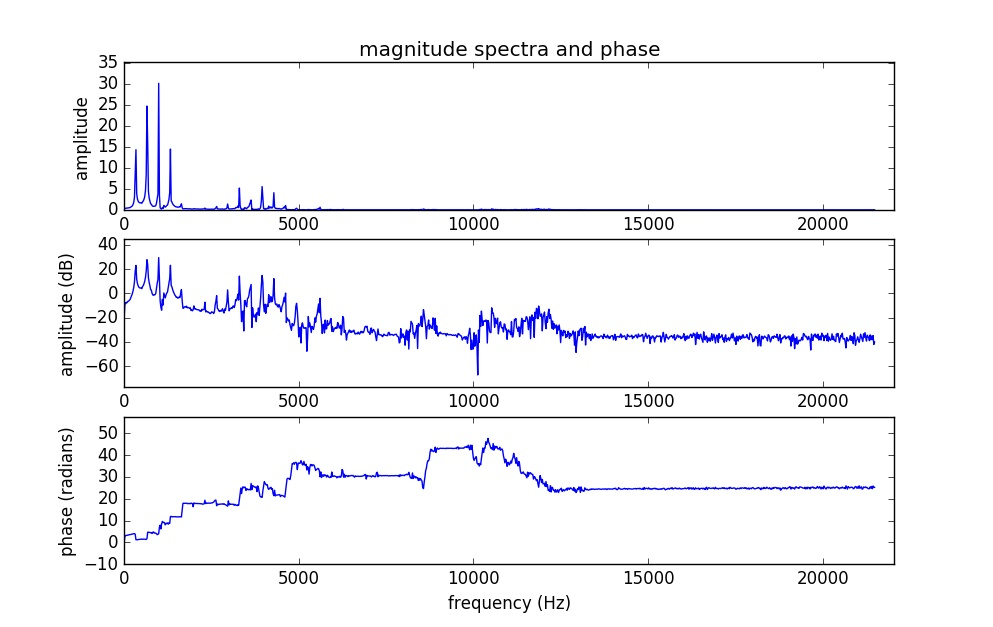# Discrete Fourier Transform

Python. This is my DFT implementation to test a wav file. It only accepts mono wav files with 44100 Hz sample rate. I used 32 floating point (with max 23 mantissa) in the range -1 and +1 for normalisation.

I chose 2048 for N points. DFT’s computational complexity is O(N^2) while FFT’s computational complexity is O(NlogN), so it takes very long time when N point is chosen too big. It is acceptable till 8192. After that, it takes time. There is also time starting variable, so it can be start anywhere from 0. I did not use windowing with zero padding at this implementation because it was a direct application of DFT:

(1)where k = 0, 1, 2,…, N – 1

The implementation gives magnitude spectra (one in normal one in dB) and phase. It is easier to see to the peaks (fundamental frequency and harmonics) in the normal spectrum, that’s why I added the normal magnitude spectrum to the figure as well. Since magnitude spectrum is symmetric, it would be better to take the first halves of the absolute value of spectra. Finally, to show the results in frequency range, frequency resolution (sample rate/N) was applied to the plots.

I used a short frame of soprano singing at E4 to test it. It is clearly to see peaks in both spectrum since it was a single note singing. The first peak is fundamental frequency. It is approximately 336 Hz. However, she was singing in vibrato style, plus it depends on choosing starting time and N values. Therefore, it is a good idea to check its harmonics and measure their average values. For example, first harmonic/2, second harmonic/3, and third harmonic/4. Then, measuring the final average value for all of them can approximately give the correct fundamental frequency she was singing.

First harmonic is 650 Hz, second harmonic is 987 Hz, and third harmonic is 1323 Hz. Average values are approximately 325, 329, and 331 Hz in order. Final average value for 4 frequencies is 330.25 Hz. E4 frequency is exactly 329.63 Hz. As a result, it is a pretty close approximation. I would finally say that she is a well trained (opera) singer.References:

 Mathematics of the Discrete Fourier Transform, Julius O. Smith III

 The Scientist and Engineer’s Guide to
Digital Signal Processing, Steven W. Smith

 The Frequency Domain, www.alwayslearn.com